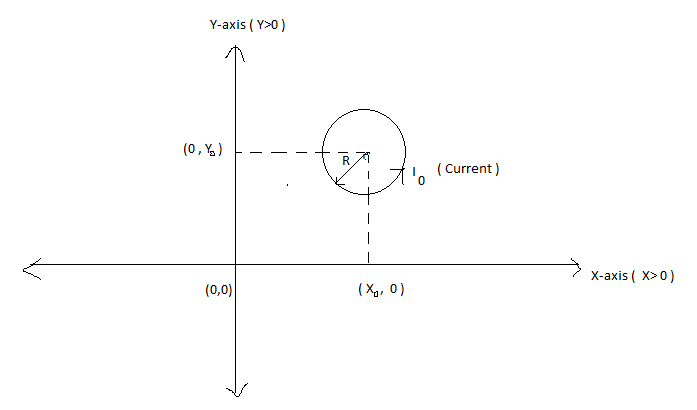# Magnetic flux + Calculus = MagaCulusLet an circular current loop is placed in X-Y co-ordinate plane such That it's centre lies on Point $P({ x }_{ 0 } ,{ y }_{ 0 })$ in First quadrant.

Let ${ I }_{ 0 }$ current is flowing in the Loop. Then find The Magnetic flux passing through The X-Y plane where X - Coordinate Has The restriction That:

$X \le 0$

Details

• $R = 1$ m
• $x_0 = 2$ m
• $y_0 = 4$ m
• $I_0 = 8 \times 10^7$ C/s
×